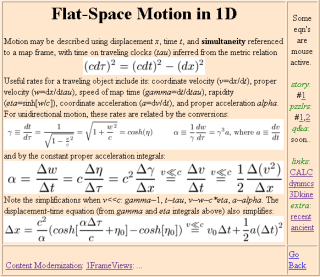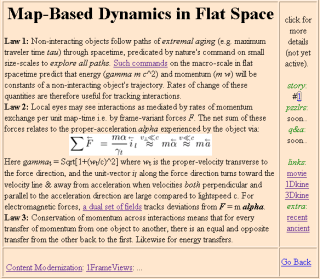# The kinematics-to-dynamics transition: story #1

[Slide 1] [Slide 2]

A bit about the modern understanding of motion and its causes may be injected into the standard intro-physics curriculum after velocity and acceleration are introduced, when Newton's laws are next on the agenda. Specifically, before students practice applying the incorrect but useful concepts of global-time and frame-invariant force inherent in Newton's original world view, some clues about the way modern views of time and space validate Newton's Laws as low-speed approximations might facilitate embrace of the classical world view with "grains of salt strategically placed" to minimize cognitive dissonance later on.

The two slides to which this story refers are executive summaries, i.e. summaries intended for folks who may not understand the concepts contained therein. Thus educators more comfortable with the linear introduction of concepts might say that one way to introduce these slides is by "engineering speak": a way to approach the onion of understanding by sampling it from all directions one layer at a time, with the intention of delving deeper on future trips around.

Depending on which examples are brought up in class, exposure to such slides should at the least serve to: (i) warn students by example that time is not a global variable even if their textbook will be treating it as so, and (ii) provide students with links to related topics that they might find of interest. It may also empower students so inclined to solve problems of high speed motion from the vantage point of the metric equation and a single frame, although this is not an expectation of this "first-encounter" storyline. Moreover single-frame approaches to spacetime preclude a full treatment of multiple-frame issues, like frame-dependent simultaneity and consideration of object sizes measured by moving yardsticks.

## Slide 1: Map-Based Motion in SpaceTimeThis slide introduces "traveler-time" and related variables for describing motion at any speed from the perspective of a single reference "map-frame" via the flat space metric equation: Minkowski's space-time version of Pythagoras' theorem. In this context it is first offered to intro-physics students as "setup" for the below slide on dynamics in spacetime, immediately following their introduction to Galilean concepts of velocity and acceleration but before the students are introduced to the classical version of Newton's laws.

The formal point of this slide is to show that time proceeds differently on traveling clocks, according to a spacetime version of Pythagoras' theorem. Moreover, once variables are introduced to take traveler-time into account, it's easy to show that the constant acceleration equations that they've been learning to use in class are low speed versions of similar (if more complicated) equations that work at any speed. The subject of modern descriptions of motion at hand, a slide on modern views of the causes of motion is a natural next step.

## Slide 2: Forces in SpaceTimeThis slide offers a modernized version of Issac Newton's three-law summary of dynamics that's useful locally* in spacetime even when curved. In this storyline, it is first offered to intro-physics students after the above slide and immediately before the students are introduced to Newton's laws.

The formal point of this slide for intro-physics students is to follow the definition of variables above with an outline of the way forces and accelerations fit into our modern understanding of motion's causes. It also indicates that the classical version of Newton's laws (to be introduced in the upcoming chapter and applied in chapters to follow) offers a simplification which works well at low speeds.

* For those more technically inclined, along with the equivalence principle's guarantee of locally-inertial frames in curved spacetime, the geodesic equation indicates that this flat-space equation applies locally in any coordinate system if we are willing to define affine-connection forces which appear by virtue of one's choice of coordinate system to act on every ounce of an object's being. Gravity on earth (i.e. in a shell-frame) is one of several classic examples of an affine-connection force. Hence at low speeds, at least, the use of such forces is well-established in practice.

This page is http://www.umsl.edu/~fraundor/help/story001.html. The person responsible for errors is P. Fraundorf. This site is hosted by the Department of Physics and Astronomy (and Center for Molecular Electronics) at UM-StL. Mindquilts site page requests ~2000/day approaching a million per year. Requests for a "stat-counter linked subset of pages" since 4/7/2005: .